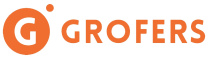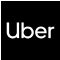New update is available. Click here to update.
Topics

# Pair Sum

Easy0/40
Average time to solve is 15m+77 more companies

## Problem statement

You are given an integer array 'ARR' of size 'N' and an integer 'S'. Your task is to return the list of all pairs of elements such that each sum of elements of each pair equals 'S'.

Note:

``````Each pair should be sorted i.e the first value should be less than or equals to the second value.

Return the list of pairs sorted in non-decreasing order of their first value. In case if two pairs have the same first value, the pair with a smaller second value should come first.
``````
Detailed explanation ( Input/output format, Notes, Images )
Constraints:
``````1 <= N <= 10^3
-10^5 <= ARR[i] <= 10^5
-2 * 10^5 <= S <= 2 * 10^5

Time Limit: 1 sec
``````
Sample Input 1:
``````5 5
1 2 3 4 5
``````
Sample Output 1:
``````1 4
2 3
``````
Explaination For Sample Output 1:
``````Here, 1 + 4 = 5
2 + 3 = 5
Hence the output will be, (1,4) , (2,3).
``````
Sample Input 2:
``````5 0
2 -3 3 3 -2
``````
Sample Output 2:
``````-3 3
-3 3
-2 2
``````Console